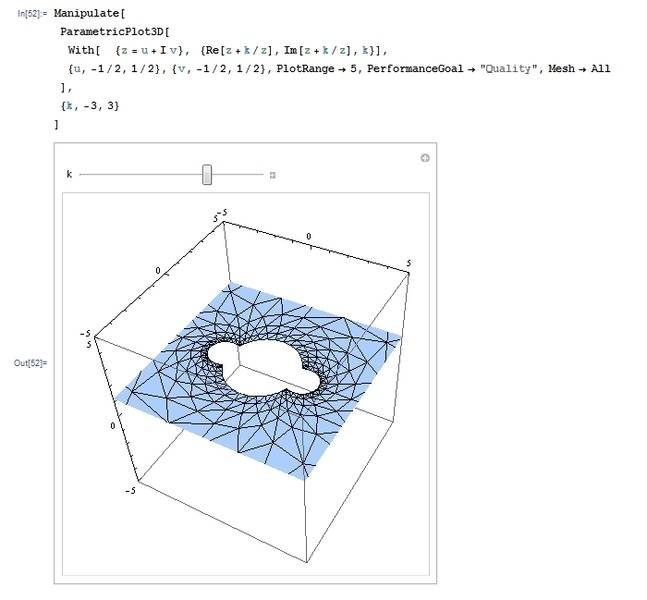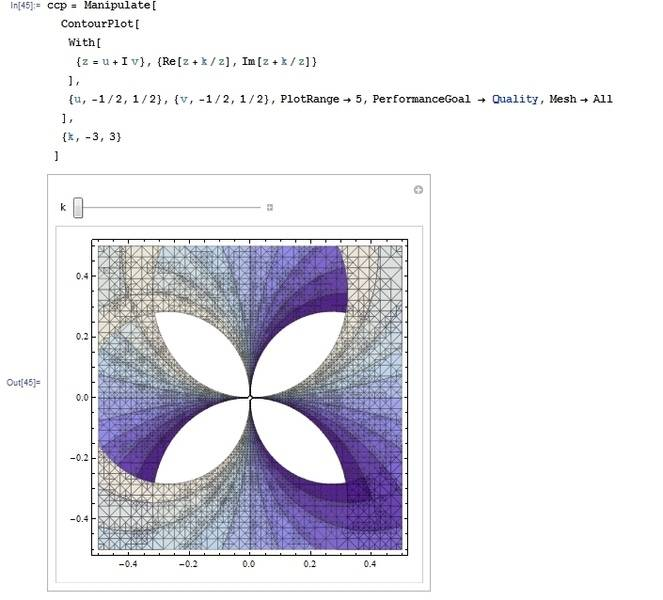# Finding Surface for Complex ParametricPlot3D

zxh
Normally, complex plot visualization is a 4D problem. Coloring re or I am separately isn't very intuitive.

Otoh, using Mathematica's ParametricPlot3d gives all the information and leaves us with the z-axis unused. Example for z→z+k/z:I'm trying to find a surface which when projected onto the xy-plane gives the iso displacement lines of the complex parametric plot. (Mathematica uses polygons for computation here).

An alternative would be to compute the grid line density for the z-value.
I looked at a density/contour plot, but it appears quite different:How would you go about this? Is this connected to riemann surfaces? It seems for higher k's the surface would intersect/overlap itself/ project non-injective.

Last edited: